Courses
Courses for Kids
Free study material
Offline Centres
More

# NCERT Exemplar for Class 7 Maths Solutions Chapter 10 Algebraic ExpressionsLast updated date: 27th Nov 2023
Total views: 422.4k
Views today: 8.22k

## NCERT Exemplar for Class 7 Maths Chapter - 10 Algebraic Expressions

Access NCERT Exemplar Solutions for Class 10 Mathematics

Chapter 10. -Algebraic Expression

(Examples, Easy Methods and Step by Step Solutions)

### Solved examples

In Examples 1 to 3, there are four options, out of which one is correct. Write the correct answer.

Example 1: The like terms in  $3x(3 - 2y)$  and  $2(xy + {x^2})$  are

a)      $9{\kern 1pt} x$  and  $2{x^2}$

b)     $- 6{\kern 1pt} x{\kern 1pt} y$  and  $2{\kern 1pt} x{\kern 1pt} y$

c)      $9{\kern 1pt} x$  and  $2{\kern 1pt} x{\kern 1pt} y$

d)     $- 6{\kern 1pt} x{\kern 1pt} y$  and  $2{\kern 1pt} {x^2}$ .

Ans: The correct answer is (b).

Example 2: The coefficient of  $x{\kern 1pt} y$  in  $3{x^2}zy + 7xyz - 2{z^2}x$  is

a)      $3{\kern 1pt} z$

b)     $- 2$

c)      $7{\kern 1pt} y{\kern 1pt} z$

d)     $7{\kern 1pt} z$

Example 3: The factors of the term  $- x{\kern 1pt} {y^2}$  are

(a)  $x \times y \times y$

(b)  $- 1 \times y \times y$

(c)  $- 1 \times x \times y$

(d)  $- 1 \times x \times y \times y$

In Examples 4 to 7, fill in the blanks to make the statements true.

Example 4: An algebraic expression having one or more terms with non-negative integral exponents of the variables is called ___________.

Ans:  Polynomials.

Example 5: Numerical factor in any term of a polynomial is called ___________ of the term.

Ans: Numerical coefficient or coefficient.

Example 6: The terms with different algebraic factors are called ______.

Ans: Unlike terms

Example 7: The terms with the same algebraic factors are called _______.

Ans: Like terms

In Examples 8 to 10, state whether the statements are True or False.

Example 8: An expression with two terms is called a binomial.

Ans: True

Example 9: Every polynomial is a monomial.

Ans: False

Example 10: The value of a variable is fixed.

Ans: False

Example 11: Twice the sum of length x and breadth y of a rectangle is the perimeter of a rectangle. Write the expression for perimeter.

Ans: Perimeter of rectangle = 2 (Length + Breadth)

= 2 (x + y) = 2x+2y

Example 12: Identify the term containing  ${u^2}$  in the expression  ${u^3} + 3{u^2}v + 3u{v^2} + {v^3}$  and write its coefficient.

Ans: Term containing  ${u^2} = 3{u^2}v$

Coefficient of  ${u^2} = 3v$

Example 13: Simplify the expression by combining the like terms:

$7{x^3} - 3{x^2}y + x{y^2} + {x^2}y - {y^3}$

Ans: Rearranging the terms in the given expression, we get

$7{x^3} - 3{x^2}y + {x^2}y + x{y^2} - {y^3}$

$= 7{x^3} + \left( { - 3{x^2}y} \right) + {x^2}y + x{y^2} - {y^3}$

[using distributive property}

$= 7{x^3} + ( - 3 + 1){x^2}y + x{y^2} - {y^3}$

$= 7{x^3} + ( - 2){x^2}y + x{y^2} - {y^3}$

$= 7{x^3} - 2{x^2}y + x{y^2} - {y^3}$

Example 14: Subtract the sum of  $- 3{x^3}{y^2} + 2{x^2}{y^3}$  and  $- 3{x^2}{y^3} - 5{y^4}$  from  ${x^4} + {x^3}{y^2} + {x^2}{y^3} + {y^4}$ .

Ans:

$\Rightarrow \left( -3{{x}^{3}}{{y}^{2}}+2{{x}^{2}}{{y}^{3}} \right)+\left( -3{{x}^{2}}{{y}^{3}}-5{{y}^{4}} \right)$

$\Rightarrow -3{{x}^{3}}{{y}^{2}}+2{{x}^{2}}{{y}^{3}}-3{{x}^{2}}{{y}^{3}}-5{{y}^{4}}$

$\Rightarrow -3{{x}^{3}}{{y}^{2}}-{{x}^{2}}{{y}^{3}}-5{{y}^{4}}$

Sum =$- 3{x^3}{y^2} - {x^2}{y^3} - 5{y^4}$

$\Rightarrow \left( {{x}^{4}}+{{x}^{3}}{{y}^{2}}+{{x}^{2}}{{y}^{3}}+{{y}^{4}} \right)-\left( -3{{x}^{3}}{{y}^{2}}-{{x}^{2}}{{y}^{3}}-5{{y}^{4}} \right)$

$\Rightarrow {{x}^{4}}+{{x}^{3}}{{y}^{2}}+{{x}^{2}}{{y}^{3}}+{{y}^{4}}+3{{x}^{3}}{{y}^{2}}+{{x}^{2}}{{y}^{3}}+5{{y}^{4}}$

$\Rightarrow {{x}^{4}}+{{x}^{3}}{{y}^{2}}+3{{x}^{3}}{{y}^{2}}+{{x}^{2}}{{y}^{3}}+{{x}^{2}}{{y}^{3}}+{{y}^{4}}+5{{y}^{4}}$

$\Rightarrow {{x}^{4}}+4{{x}^{3}}{{y}^{2}}+2{{x}^{2}}{{y}^{3}}+6{{y}^{4}}$

Difference = ${x^4} + 4{x^3}{y^2} + 2{x^2}{y^3} + 6{y^4}$

Example 15: Find the value of the following expressions at  $a = 1$  and  $b = - 2$  :

i.       ${a^2} + {b^2} + 3ab$

ii.       ${a^3} + {a^2}b + a{b^2} + {b^3}$

Ans: (i) Value of  ${a^2} + {b^2} + 3ab$  at  $a = 1$  and  $b = - 2$

$= {(1)^2} + {( - 2)^2} + 3(1)( - 2)$

$= 1 + 4 - 6$

$= 5 - 6$

$= - 1$

(ii) Value of  ${a^3} + {a^2}b + a{b^2} + {b^3}$  at  $a = 1$  and  $b = - 2$

$= {(1)^3} + {(1)^2}( - 2) + (1){( - 2)^2} + {( - 2)^3}$

$= 1 - 2 + 4 - 8$

$= 5 - 10$

$= - 5$

In each of the questions 1 to 16, out of the four options, only one is correct. Write the correct answer.

1. An algebraic expression containing three terms is called a

1. Monomial

2. Binomial

3. Trinomial

4. All of these

Ans: c) Trinomial

An algebraic expression having one term is called monomial. An algebraic expression containing two unlike terms is called binomial and an algebraic expression containing three unlike terms is called trinomial.

2. Number of terms in the expression $3{x^2}y - 2{y^2}z - {z^2}x + 5$ is

1. 2

2. 3

3. 4

4. 5

Ans: c) 4

In the expression $3{x^2}y - 2{y^2}z - {z^2}x + 5$, We have four terms which are $3{x^2}y$, $- 2{y^2}z$, $- {z^2}x$ and 5.

3. The terms of expression $4{x^2} - 3xy$ are

1. $4{x^2}$ and $- 3xy$

2. $4{x^2}$ and $3xy$

3. $4{x^2}$ and $- xy$

4. ${x^2}$ and $xy$

Ans: a) $4{x^2}$ and $- 3xy$

The terms in the given expression $4{x^2} - 3xy$ are $4{x^2}$ and $- 3xy$.

4. Factors of   $- 5{x^2}{y^2}z$   are

1.   $- 5 \times x \times y \times z$

2.   $- 5 \times {x^2} \times y \times z$

3.   $- 5 \times x \times x \times y \times y \times z$

4.   $- 5 \times x \times y \times {z^2}$

Ans: c)   $- 5 \times x \times x \times y \times y \times z$

$- 5{x^2}{y^2}z$   can be written as   $- 5 \times x \times x \times y \times y \times z$

5. Coefficient of   $x$   in   $- 9x{y^2}z$   is

1.   $9yz$

2.   $- 9yz$

3.   $- 9{y^2}z$

4.   $- 9{y^2}z$

Ans: d)   $- 9{y^2}z$

Coefficient of x in   $- 9x{y^2}z$   is   $- 9{y^2}z$

6. Which of the following is a pair of like terms?

1. $- 7x{y^2}z, - 7{x^2}yz$

2. $- 10xy{z^2},3xy{z^2}$

3. $3xyz,3{x^2}{y^2}{z^2}$

4. $4xy{z^2},4{x^2}yz$

Ans: b)$- 10xy{z^2},3xy{z^2}$

Like terms are the terms whose variables and their exponents are the same. In the given options only option b has the terms whose variables and exponents are the same.

7. Identify the binomial out of the following:

1. $3x{y^2} + 5y - {x^2}y$

2. ${x^2}y - 5y - {x^2}y$

3. $xy + yz + zx$

4. $3x{y^2} + 5y - x{y^2}$

Ans: d) $3x{y^2} + 5y - x{y^2}$

An algebraic expression containing two unlike terms is called binomial. Grouping the like terms, we have $3x{y^2} + 5y - x{y^2} = 3x{y^2} - x{y^2} + 5y = 2x{y^2} + 5y$. Here we only have two terms, hence the option d is correct.

8. The sum of   ${x^4} - xy + 2{y^2}$   and   $- {x^4} + xy + 2{y^2}$   is

1. Monomial and polynomial in   $y$

2. Binomial and Polynomial

3. Trinomial and polynomial

4. Monomial and polynomial in   $x$

Ans: a) Monomial and polynomial in y.

First find the sum of   ${x^4} - xy + 2{y^2}$   and   $- {x^4} + xy + 2{y^2}$

That is   $= \left( {{x^4} - xy + 2{y^2}} \right) + \left( { - {x^4} + xy + 2{y^2}} \right)$

$= {x^4} - xy + 2{y^2} - {x^4} + xy + 2{y^2}$

$= {x^4} - {x^4} - xy + xy + 2{y^2} + 2{y^2}$     {grouping like terms}

$= 0 + 0 + 4{y^2}$

$= 4{y^2}$

Here we can see that the obtained term is monomial and it is a polynomial in y.

9. The subtraction of 5 times of   $y$   from   $x$   is

1.   $5x - y$

2.   $y - 5x$

3.   $x - 5y$

4.   $5y - x$

Ans: c)  $5y - x$

5 times of y means   $5y$

The subtraction of 5 times of y from x means    $5y - x$

10.   $- b - 0$   is equal to

1.   $- 1 \times b$

2.   $1 - b - 0$

3.   $0 - ( - 1) \times b$

4.   $- b - 0 - 1$

Ans: a)   $- 1 \times b$

Because the value of   $- b - 0$   is   $- b$  . That is   $- b - 0 = - b$

Now we have to check for all the given options

1.   $- 1 \times b = - b$

2.   $1 - b - 0 = 1 - b$

3.   $0 - \left( { - 1} \right) \times b = 1b$

4.   $- b - 0 - 1 = - b - 1$

Hence option a) is correct.

11. The side length of the top of the square table is   $x$  . The expression for perimeter is:

1.   $4 + x$

2.   $2x$

3.   $4x$

4.   $8x$

Ans: c)   $4x$

We know that the perimeter of a square is 4 times its side.

Since they are given that the side of a square table is x.

Therefore, perimeter of a square table is   $4x$

12. The number of scarfs of length half meter that can be made from   $y$   meters of cloth is:

1.   $2y$

2.   $\dfrac{y}{2}$

3.   $y + 2$

4.   $y + \dfrac{1}{2}$    $4x$

Ans: a) 2y

They have given that the length if scarf is half meter   $\Rightarrow \dfrac{1}{2}m$

So, the number of scarf’s which can be made from y meters is

$\Rightarrow y \div \left( {\dfrac{1}{2}} \right)$

$\Rightarrow y \times \dfrac{2}{1}$

$\Rightarrow 2y$

Hence option a) is correct.

13.   $123{x^2}y - 138{x^2}y$   is a like term of:

1.   $10xy$

2.   $- 15xy$

3.   $- 15x{y^2}$

4.   $10{x^2}y$

Ans: d)   $10{x^2}y$

Given   $123{x^2}y - 138{x^2}y$  . We can see that the two terms are like terms and we can subtract them.

Then   $123{x^2}y - 138{x^2}y = - 15{x^2}y$  . We know that like terms are the terms whose variables and their exponents are the same.

If we observe the options the correct option is d).

14. The value of   $3{x^2} - 5x + 3$   when   $x = 1$   is

1. 1

2. 0

3.   $- 1$

4. 11

Ans: a) 1

Given   $3{x^2} - 5x + 3$  .

Put   $x = 1$   in the given equation.

$= 3{\left( 1 \right)^2} - 5\left( 1 \right) + 3$

$= 3 - 5 + 3$

$= 6 - 5$

$= 1$

Hence the correct option is a).

15. The expression for the number of diagonals that we can make from one vertex of a   $n$   sided polygon is:

1.   $2n + 1$

2.   $n - 2$

3.   $5n + 2$

4.   $n - 3$

Ans: d)   $n - 3$

We know that the vertex is formed by joining the two sides.

Diagonal is line segment joining the two opposite vertices. So, the number of diagonal formed by one vertex is   $n - 3$

16. The length of a side of a square is given as   $2x + 3$  . Which expression represents the perimeter of the square?

1.   $2x + 16$

2.   $6x + 9$

3.   $8x + 3$

4.   $8x + 12$

Ans: d)   $8x + 12$

We know that the perimeter of a square is 4 times its side.

That is the perimeter of a square   $P = 4 \times \left( {{\text{side}}} \right)$

Given the side is   $2x + 3$

$\Rightarrow P = 4 \times \left( {2x + 3} \right)$

$\Rightarrow P = 8x + 12$

Hence the option d) is correct.

In questions 17 to 32, fill in the blanks to make the statements true.

17. Sum or difference of two like terms is ________.

Ans: Sum or difference of two like terms is like terms.

For example, take any terms like   $xy$   and   $5xy$  .

Then   $xy + 5xy = 6xy$

$xy - 5xy = - 4xy$   is a similar term.

18. In the formula, area of circle   $= \pi {r^2}$  , the numerical constant of the expression   $\pi {r^2}$   is ________.

Ans: In the formula, area of circle   $= \pi {r^2}$  , the numerical constant of the expression   $\pi {r^2}$   is   $\pi$  .

Because the value of ‘r’ that is radius will change but not   $\pi$  . The value of   $\pi = \dfrac{{22}}{7}$  . It is a constant.

19.   $3{a^2}b$   and   $- 7b{a^2}$   are ________ terms.

Ans:   $3{a^2}b$   and   $- 7b{a^2}$   are like terms.

Because in both terms the variables are the same and the exponents of the variables in the two terms are the same.

20.   $- 5{a^2}b$  and $- 5{b^2}a$ are ________ terms.

Ans:   $- 5{a^2}b$   and   $- 5{b^2}a$   are unlike terms.

Because in both terms the variables are the same, but the exponents of the variables in the two terms are different.

21. In the expression   $2\pi r$  , the algebraic variable is ________.

Ans: In the expression   $2\pi r$  , the algebraic variable is ‘r’.

Because the value of ‘r’ that is radius will change but not   $\pi$  . The value of   $\pi = \dfrac{{22}}{7}$  . It is a constant.

22. Number of terms in a monomial is ________.

Ans: Number of terms in a monomial is one.

23. Like terms in the expression   $n(n + 1) + 6(n - 1)$   are ___________and ________.

Ans: Like terms in the expression   $n\left( {n + 1} \right) + 6\left( {n - 1} \right)$  are n and 6n.

Because   $n\left( {n + 1} \right) + 6\left( {n - 1} \right) = {n^2} + n + 6n - 6$  , we can see that only n and 6n are like terms.

24. The expression   $13 + 90$   is a ________.

Ans: The expression   $13 + 90$   is a constant.

Because   $13 + 90 = 103$   and 103 is a constant.

25. The speed of the car is 55 km/hrs. The distance covered in y hours is ________.

Ans: Given, the speed of the car is 55 km/hrs. The distance covered in y hours is 55y km.

We know that the formula of distance is   ${\text{Distance}} = {\text{speed}} \times {\text{time}}$

Therefore, distance covered in y hour is   $55 \times y\,\,km = 55y\,\,km$

26.   $x + y + z$   is an expression which is neither monomial nor ________.

Ans:   $x + y + z$   is an expression which is neither monomial nor binomial.

Because in   $x + y + z$  , we can see that there are 3 unlike terms, hence it is trinomial.

27. If   $\left( {{x^2}y + {y^2} + 3} \right)$   is subtracted from $\left( {3{x^2}y + 2{y^2} + 5} \right)$, then coefficient of y in the result ________.

Ans: As per the given question, we have to subtract   $\left( {{x^2}y + {y^2} + 3} \right)$   from $\left( {3{x^2}y + 2{y^2} + 5} \right)$

That is

$= \left( {3{x^2}y + 2{y^2} + 5} \right) - \left( {{x^2}y + {y^2} + 3} \right)$

$= 3{x^2}y + 2{y^2} + 5 - {x^2}y - {y^2} - 3$

$= 3{x^2}y - {x^2}y + 2{y^2} - {y^2} + 5 - 3$

$= 2{x^2}y + {y^2} + 2$

Therefore, the coefficient of y is $2{x^2}$.

28.   $- a - b - c$   is same as   $- a - \left( {\_\_\_\_\_\_\_\_} \right)$

Ans: We have   $- a - b - c$  , Now taking   $-$   (minus) common in the last two terms, then

$- a - b - c = - a - \left( {b + c} \right)$

So,   $- a - b - c$   is same as   $- a - \left( {b + c} \right)$

29. The unlike terms in perimeters of following figures are___________ and __________.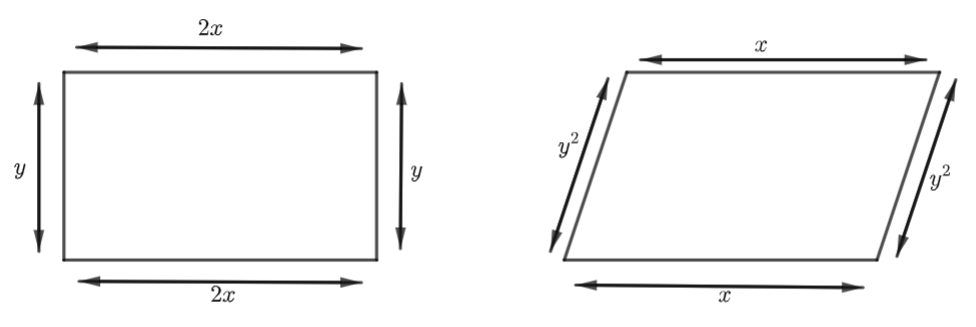Ans: We know that the perimeter is the sum of all sides of the given figure.

Now in figure (i) if we add all the sides we will get the perimeter.

Perimeter = sum of all sides

$= 2x + y + 2x + y$

$= 4x + 2y$

That is the perimeter of figure (i) is   $4x + 2y$  .

Now perimeter of figure (ii) is

Perimeter = sum of all sides

$= x + {y^2} + x + {y^2}$

$= 2x + 2{y^2}$

That is the perimeter of figure (ii) is   $2x + 2{y^2}$  .

We can observe that the unlike terms in the perimeters of two figures are   $2y$   and   $2{y^2}$

30. On adding a monomial _____________ to   $- 2x + 4{y^2} + z$  , the resulting expression becomes a binomial

Ans: We know that monomial means an algebraic expression containing a single term. If we add   $2x$  ,   $- 4{y^2}$   and   $- z$   to the given expression then only the obtained result will be a binomial expression.

$\Rightarrow 2x + \left( { - 2x + 4{y^2} + z} \right) = 2x - 2x + 4{y^2} + z = 4{y^2} + z$

$\Rightarrow - 4{y^2} + \left( { - 2x + 4{y^2} + z} \right) = - 4{y^2} - 2x + 4{y^2} + z = - 2x + z$

$\Rightarrow - z + \left( { - 2x + 4{y^2} + z} \right) = - z - 2x + 4{y^2} + z = - 2x + 4{y^2}$.

31.   $3x + 23{x^2} + 6{y^2} + 2x + {y^2} + \_\_\_\_\_\_\_\_\_\_\_ = 5x + 7{y^2}$  .

Ans: Let's consider that the required result will be ‘M’.

Then

$\Rightarrow 3x + 23{x^2} + 6{y^2} + 2x + {y^2} + M = 5x + 7{y^2}$

$\Rightarrow M = 5x + 7{y^2} - \left( {3x + 23{x^2} + 6{y^2} + 2x + {y^2}} \right)$

$\Rightarrow M = 5x + 7{y^2} - 3x - 23{x^2} - 6{y^2} - 2x - {y^2}$

$\Rightarrow M = 5x - 3x - 2x - 6{y^2} + 7{y^2} - {y^2} - 23{x^2}$

$\Rightarrow M = - 23{x^2}$  .

Hence,   $- 23{x^2}$   is the required result.

32. If Rohit has 5xy toffees and Shantanu has 20yx toffees, then Shantanu has _____ more toffees.

Ans:

Given, Rohit has 5xy toffees.

Shantanu has 20yx toffees.

Then the difference is   $20xy - 5xy = 15xy$  .

Hence shantanu has 15xy more toffees.

In questions 33 to 52, state whether the statements given are True or False.

33.   $1 + \dfrac{x}{2} + {x^3}$   is a polynomial.

Ans: True.

Because we know that a polynomial means an algebraic expression which contains more than one term. In the given expression we have 3 terms.

34.   $(3a - b + 3) - (a + b)$   is a binomial.

Ans: False.

Because   $(3a - b + 3) - (a + b) = 3a - b + 3 - a - b$

$(3a - b + 3) - (a + b) = 2a - 2b + 3$

We can see that it has 3 terms and it is trinomial.

35. A trinomial can be a polynomial.

Ans: True.

Because a polynomial is an algebraic expression which contains more than one term and trinomial means an algebraic expression which contains 3 unlike terms.

36. A polynomial with more than two terms is a trinomial.

Ans: False.

Because a polynomial with more than two terms is called a binomial.

37. Sum of x and y is   $x + y$  .

Ans: True.

Because the sum of x and y is   $x + y$   only.

38. Sum of 2 and p is 2p.

Ans: False.

Because the sum of 2 and p is   $2 + p$   and the product of 2 and p is 2p.

39. A binomial has more than two terms.

Ans: False.

Because a binomial has more than two terms will become trinomial and polynomial. A binomial contains only two unlike terms.

40. A trinomial has exactly three terms.

Ans: True.

Because trinomial is an algebraic expression which contains 3 unlike terms.

41. In like terms, variables and their powers are the same.

Ans: True.

Because the variables and their powers we call them algebraic factors and they are same in case of like terms.

42. The expression   $x + y + 5x$   is a trinomial.

Ans: False.

Because we know that a trinomial contains three unlike terms. In the given expression we have like terms.

$\Rightarrow x + y + 5x = x + 5x + y = 6x + y$  . We have 2 terms and it is binomial.

43. 4p is the numerical coefficient of   ${q^2}$   in   $- 4p{q^2}$  .

Ans: False.

Because we know that a numerical coefficient is any constant term in a given algebraic expression. Here the numerical coefficient is -4.

44.   $5a$  and   $5b$   are unlike terms.

Ans: True.

Because in two terms we have different variables.

45. Sum of   ${x^2} + x$   and   $y + {y^2}$   is   $2{x^2} + 2{y^2}$

Ans: False.

Because,

$= \left( {{x^2} + x} \right) + \left( {y + {y^2}} \right)$

$= {x^2} + x + y + {y^2}$   (Because all terms are unlike terms).

46. Subtracting a term from a given expression is the same as adding its additive inverse to the given expression.

Ans: True.

Because the additive inverse of the expression is the same as the subtracting the term.

47. The total number of planets of the Sun can be denoted by the variable n.

Ans:  False.

Because, the total number of planets of the Sun is 8 and which is a constant not a variable.

48. In like terms, the numerical coefficients should also be the same.

Ans: False.

Because, while identifying the like terms we observe whether the terms have the same variable and the same powers of variable.

49. If we add a monomial and binomial, then the answer can never be a monomial.

Ans: False.

Because if we add a monomial and binomial then the obtained result can be monomial also. For example

We know that   $- x$   is a monomial and   $x + y$   is binomial. If we add these two we will have,   $\Rightarrow - x + x + y = y$   which is a monomial.

50. If we subtract a monomial from a binomial, then the answer is at least a binomial.

Ans: False.

The result will also yield monomials. For example

We know that   $x$   is a monomial and   $x + y$   is binomial. If we subtract these two, we will have, $\Rightarrow x - \left( {x + y} \right) = x - x - y = - y$ which is a monomial.

51. When we subtract a monomial from a trinomial, then the answer can be a polynomial.

Ans: True.

Because we know that   $x$   is monomial and   $x + y + z$  is a trinomial.

If we subtract one from other, we will have

$\Rightarrow x - \left( {x + y + z} \right) = x - x - y - z = - y - z$, which is a binomial.

Thus, if we subtract a monomial from a trinomial, the obtained expression will have at least two terms. By the definition of polynomial, the above statement is true.

52. When we add a monomial and a trinomial, then the answer can be a monomial.

Ans: False.

Because when we add a monomial and a trinomial, the obtained result will have at least two terms.

53. Write the following statements in the form of algebraic expressions and write whether it is monomial, binomial or trinomial.

a) x is multiplied by itself and then added to the product of x and y.

Ans: x multiplied by itself means   $x \times x = {x^2}$

Product of x and y means   $x \times y = xy$  .

x is multiplied by itself and then added to the product of x and y means

${x^2} + xy$  , the expression is binomial.

b) Three times of p and two times of q are multiplied and then subtracted from r.

Ans: Three times of p means    $3 \times p = 3p$

Two times of q means   $2 \times q = 2q$

Three times of p and two times of q are multiplied means   $3p \times 2q = 6pq$  .

Three times of p and two times of q are multiplied and then subtracted from r means   $r - 6pq$  , it is a binomial expression.

c) Product of p, twice of q and thrice of r.

Ans: Twice of q means   $2 \times q = 2q$

Thrice of r means   $3 \times r = 3r$

Product of p, twice of q and thrice of r means   $p \times 2q \times 3r = 6pqr$  , it is a monomial expression.

d) Sum of the products of a and b, b and c and c and a.

Ans: products of a and b means $a \times b = ab$.

Similarly, products of b and c and c and a means $b \times c = bc$, $c \times a = ca$.

Sum of the products of a and b, b and c and c and a means $ab + ba + ca$, it is a trinomial expression.

e) Perimeter of an equilateral triangle of side x.

Ans: We know that the perimeter of an equilateral triangle is 3 times it’s sides. Because all sides are equal in equilateral triangles.

Since the side is ‘x’ then the perimeter of an equilateral triangle is 3x, it is a monomial.

f) Perimeter of a rectangle with length p and breadth q.

Ans: We know that the perimeter of a rectangle with length p and breadth q is $2\left( {p + q} \right)$

That is  $2p + 2q$, it is a binomial expression.

g) Area of a triangle with base m and height n.

Ans: Area of a triangle with base m and height n is $\Rightarrow \dfrac{1}{2}mn$, it is a monomial expression.

h) Area of a square with side x.

Ans: Area of the square with side x is  $\Rightarrow {x^2}$, it is a monomial expression.

i) Cube of s subtracted from cube of t.

Ans: Cube of s $\Rightarrow {s^3}$

Cube of t $\Rightarrow {t^3}$

Cube of s subtracted from cube of t means ${t^3} - {s^3}$

j) Quotient of x and 15 multiplied by x.

Ans: Quotient of x and 15 is $\dfrac{x}{{15}}$.

Quotient of x and 15 multiplied by x is $\dfrac{x}{{15}} \times x = \dfrac{{{x^2}}}{{15}}$, it is a monomial expression.

k) The sum of the square of x and cube of z.

Ans: The sum of the square of x and cube of z is given by ${x^2} + {z^3}$, it is a binomial expression.

l) Two times q subtracted from cube of q

Ans: Two times q means 2q.

Two times q subtracted from the cube of q means ${q^3} - 2q$, it is a binomial expression.

54. Write the coefficient of   ${x^2}$   in the following:

a) ${x^2} - x + 4$

Ans: Coefficient of   ${x^2}$   in   ${x^2} - x + 4$   is 1

b) ${x^3} - 2{x^2} + 3x + 1$

Ans: Coefficient of   ${x^2}$   in   ${x^3} - 2{x^2} + 3x + 1$   is -2.

c) $1 + 2x + 3{x^2} + 4{x^3}$

Ans: Coefficient of   ${x^2}$   in   $1 + 2x + 3{x^2} + 4{x^3}$   is 3

d) $y + {y^2}x + {y^3}{x^2} + {y^4}{x^3}$

Ans: Coefficient of   ${x^2}$   in   $y + {y^2}x + {y^3}{x^2} + {y^4}{x^3}$   is ${y^3}$.

55. Find the numerical coefficient of each of the terms:

a) ${x^3}{y^2}z,x{y^2}{z^3}, - 3x{y^2}{z^3},5{x^3}{y^2}z, - 7{x^2}{y^2}{z^2}$

Ans: Numerical coefficient of   ${x^3}{y^2}z$   is 1

Numerical coefficient of   $x{y^2}{z^3}$   is 1

Numerical coefficient of   $- 3x{y^2}{z^3}$   is -3

Numerical coefficient of   $5{x^3}{y^2}z$   is 5

Numerical coefficient of   $- 7{x^2}{y^2}{z^2}$   is -7

b) $10xyz, - 7x{y^2}z, - 9xyz,2x{y^2}z,2{x^2}{y^2}{z^2}$

Ans: Numerical coefficient of   $10xyz$   is 10

Numerical coefficient of   $- 7x{y^2}z$   is -7

Numerical coefficient of   $- 9xyz$   is -9

Numerical coefficient of   $2x{y^2}z$   is 2

Numerical coefficient of   $2{x^2}{y^2}{z^2}$   is 2

56. Simplify the following by combining the like terms and then write whether the expression is a monomial, a binomial or a trinomial.

a) $3{x^2}y{z^2} - 3x{y^2}z + {x^2}y{z^2} + 7x{y^2}z$

Ans: Grouping the like terms and applying the mathematical operation,

$= 3{x^2}y{z^2} - 3x{y^2}z + {x^2}y{z^2} + 7x{y^2}z$

$= 3{x^2}y{z^2} + {x^2}y{z^2} - 3x{y^2}z + 7x{y^2}z$

$= 4{x^2}y{z^2} + 4x{y^2}z$

$\Rightarrow 3{x^2}y{z^2} - 3x{y^2}z + {x^2}y{z^2} + 7x{y^2}z = 4{x^2}y{z^2} + 4x{y^2}z$

It is a binomial expression.

b) ${x^4} + 3{x^3}y + 3{x^2}{y^2} - 3{x^3}y - 3x{y^3} + {y^4} - 3{x^2}{y^2}$

Ans: Grouping the like terms and applying the mathematical operation,

$= {x^4} + 3{x^3}y + 3{x^2}{y^2} - 3{x^3}y - 3x{y^3} + {y^4} - 3{x^2}{y^2}$

$= {x^4} + 3{x^3}y - 3{x^3}y + 3{x^2}{y^2} - 3{x^2}{y^2} - 3x{y^3} + {y^4}$

$= {x^4} - 3x{y^3} + {y^4}$

$\Rightarrow {x^4} + 3{x^3}y + 3{x^2}{y^2} - 3{x^3}y - 3x{y^3} + {y^4} - 3{x^2}{y^2} = {x^4} - 3x{y^3} + {y^4}$  , it is trinomial expression.

c) ${p^3}{q^2}r + p{q^2}{r^3} + 3{p^2}q{r^2} - 9{p^2}q{r^2}$

Ans: Grouping the like terms and applying the mathematical operation,

$= {p^3}{q^2}r + p{q^2}{r^3} + 3{p^2}q{r^2} - 9{p^2}q{r^2}$

$= {p^3}{q^2}r + p{q^2}{r^3} - 6{p^2}q{r^2}$

$\Rightarrow {p^3}{q^2}r + p{q^2}{r^3} + 3{p^2}q{r^2} - 9{p^2}q{r^2} = {p^3}{q^2}r + p{q^2}{r^3} - 6{p^2}q{r^2}$  , it is a trinomial expression.

d) $2a + 2b + 2c - 2a - 2b - 2c - 2b + 2c + 2a$

Ans: Grouping the like terms and applying the mathematical operation,

$= 2a + 2b + 2c - 2a - 2b - 2c - 2b + 2c + 2a$

$= 2a + 2a - 2a + 2b - 2b - 2b + 2c - 2c + 2c$

$= 2a - 2b + 2c$

$2a + 2b + 2c - 2a - 2b - 2c - 2b + 2c + 2a = 2a - 2b + 2c$  , it is a trinomial expression.

e) $50{x^3} - 21x + 107 + 41{x^3} - x + 1 - 93 + 71x - 31{x^3}$

Ans: Grouping the like terms and applying the mathematical operation,

$= 50{x^3} - 21x + 107 + 41{x^3} - x + 1 - 93 + 71x - 31{x^3}$

$= 50{x^3} + 41{x^3} - 31{x^3} - 21x - x + 71x + 107 + 1 - 93$

$= 60{x^3} + 49x + 15$  , it is a trinomial expression.

a) ${p^2} - 7pq - {q^2}$   and   $- 3{p^2} - 2pq + 7{q^2}$

Ans:

$= \left( {{p^2} - 7pq - {q^2}} \right) + \left( { - 3{p^2} - 2pq + 7{q^2}} \right)$

$= {p^2} - 7pq - {q^2} - 3{p^2} - 2pq + 7{q^2}$

$= {p^2} - 3{p^2} - 7pq - 2pq - {q^2} + 7{q^2}$

$= - 2{p^2} - 9pq + 6{q^2}$

b) ${x^3} - {x^2}y - x{y^2} - {y^3}$   and   ${x^3} - 2{x^2}y + 3x{y^2} + 4y$

Ans:

$= \left( {{x^3} - {x^2}y - x{y^2} - {y^3}} \right) + \left( {{x^3} - 2{x^2}y + 3x{y^2} + 4y} \right)$

$= {x^3} - {x^2}y - x{y^2} - {y^3} + {x^3} - 2{x^2}y + 3x{y^2} + 4y$

$= {x^3} + {x^3} - {x^2}y - 2{x^2}y - x{y^2} + 3x{y^2} - {y^3} + 4y$

$= 2{x^3} - 3{x^2}y + 2x{y^2} - {y^3} + 4y$

c) $ab + bc + ca$   and   $- bc - ca - ab$

Ans:

$= \left( {ab + bc + ca} \right) + \left( { - bc - ca - ab} \right)$

$= ab + bc + ca - bc - ca - ab$

$= ab - ab + bc - bc + ca - ca$

$= 0$

d) ${p^2} - q + r,{q^2} - r + p$   and   ${r^2} - p + q$

Ans:

$= \left( {{p^2} - q + r} \right) + \left( {{q^2} - r + p} \right) + \left( {{r^2} - p + q} \right)$

$= {p^2} - q + r + {q^2} - r + p + {r^2} - p + q$

$= {p^2} - q + q + r - r + p - p + {r^2} + {q^2}$

$= {p^2} + {r^2} + {q^2}$

e) ${x^3}{y^2} + {x^2}{y^3} + 3{y^4}$   and   ${x^4} + 3{x^2}{y^3} + 4{y^4}$

Ans:

$= \left( {{x^3}{y^2} + {x^2}{y^3} + 3{y^4}} \right) + \left( {{x^4} + 3{x^2}{y^3} + 4{y^4}} \right)$

$= {x^3}{y^2} + {x^2}{y^3} + 3{y^4} + {x^4} + 3{x^2}{y^3} + 4{y^4}$

$= {x^3}{y^2} + {x^2}{y^3} + 3{x^2}{y^3} + 3{y^4} + 4{y^4} + {x^4}$

$= {x^3}{y^2} + 4{x^2}{y^3} + 7{y^4} + {x^4}$

f) ${p^2}qr + p{q^2}r + pq{r^2}$   and   $- 3p{q^2}r - 2pq{r^2}$

Ans:

$= \left( {{p^2}qr + p{q^2}r + pq{r^2}} \right) + \left( { - 3p{q^2}r - 2pq{r^2}} \right)$

$= {p^2}qr + p{q^2}r + pq{r^2} - 3p{q^2}r - 2pq{r^2}$

$= {p^2}qr + p{q^2}r - 3p{q^2}r + pq{r^2} - 2pq{r^2}$

$= {p^2}qr - 2p{q^2}r - pq{r^2}$

g) $uv - vw,vw - wu$   and   $wu - uv$

Ans:

$= \left( {uv - vw} \right) + \left( {vw - wu} \right) + \left( {wu - uv} \right)$

$= uv - vw + vw - wu + wu - uv$

$= uv - uv - vw + vw - wu + wu$

$= 0$

h) ${a^2} + 3ab - bc,{b^2} + 3bc - ca$   and   ${c^2} + 3ca - ab$

Ans:

$= \left( {{a^2} + 3ab - bc} \right) + \left( {{b^2} + 3bc - ca} \right) + \left( {{c^2} + 3ca - ab} \right)$

$= {a^2} + 3ab - bc + {b^2} + 3bc - ca + {c^2} + 3ca - ab$

$= {a^2} + {b^2} + {c^2} + 3ab - ab - bc + 3bc - ca + 3ca$

$= {a^2} + {b^2} + {c^2} + 2ab + 2bc + 2ca$

i) $\dfrac{5}{8}{p^4} + 2{p^2} + \dfrac{5}{8};\dfrac{1}{8} - 17p + \dfrac{9}{8}{p^2}$   and   ${p^5} - {p^3} + 7$

Ans:

$= \left( {\dfrac{5}{8}{p^4} + 2{p^2} + \dfrac{5}{8}} \right) + \left( {\dfrac{1}{8} - 17p + \dfrac{9}{8}{p^2}} \right) + \left( {{p^5} - {p^3} + 7} \right)$

$= \dfrac{5}{8}{p^4} + 2{p^2} + \dfrac{5}{8} + \dfrac{1}{8} - 17p + \dfrac{9}{8}{p^2} + {p^5} - {p^3} + 7$

$= {p^5} + \dfrac{5}{8}{p^4} - {p^3} + 2{p^2} + \dfrac{9}{8}{p^2} - 17p + \dfrac{5}{8} + \dfrac{1}{8} + 7$

$= {p^5} + \dfrac{5}{8}{p^4} - {p^3} + \dfrac{{16{p^2} + 9{p^2}}}{8} - 17p + \dfrac{{5 + 1 + 56}}{8}$

$= {p^5} + \dfrac{5}{8}{p^4} - {p^3} + \dfrac{{25{p^2}}}{8} - 17p + \dfrac{{62}}{8}$

j) $t - {t^2} - {t^3} - 14;\quad 15{t^3} + 13 + 9t - 8{t^2};\quad 12{t^2} - 19 - 24t$   and   $4t - 9{t^2} + 19{t^3}$

Ans:

$= \left( {t - {t^2} - {t^3} - 14} \right) + \left( {15{t^3} + 13 + 9t - 8{t^2}} \right) + \left( {12{t^2} - 19 - 24t} \right) + \left( {4t - 9{t^2} + 19{t^3}} \right)$

$= t - {t^2} - {t^3} - 14 + 15{t^3} + 13 + 9t - 8{t^2} + 12{t^2} - 19 - 24t + 4t - 9{t^2} + 19{t^3}$

$= t - 24t + 4t + 9t - {t^2} - 8{t^2} + 12{t^2} - 9{t^2} + 19{t^3} + 15{t^3} - {t^3} + 13 - 19 - 14$

$= t - 24t + 4t + 9t - {t^2} - 8{t^2} + 12{t^2} - 9{t^2} + 19{t^3} + 15{t^3} - {t^3} + 13 - 19 - 14$

$= 10t - 6{t^2} + 33{t^3} - 20$

58. Subtract

a) $- 7{p^2}qr$   from   $- 3{p^2}qr$  .

Ans:

$= \left( { - 3{p^2}qr} \right) - \left( { - 7{p^2}qr} \right)$

$= - 3{p^2}qr + 7{p^2}qr$

$= 4{p^2}qr$

b) $- {a^2} - ab$   from   ${b^2} + ab$  .

Ans:

$= \left( {{b^2} + ab} \right) - \left( { - {a^2} - ab} \right)$

$= {b^2} + ab + {a^2} + ab$

$= {a^2} + {b^2} + 2ab$

c) $- 4{x^2}y - {y^3}$   from   ${x^3} + 3x{y^2} - {x^2}y$  .

Ans:

$= \left( {{x^3} + 3x{y^2} - {x^2}y} \right) - \left( { - 4{x^2}y - {y^3}} \right)$

$= {x^3} + 3x{y^2} - {x^2}y + 4{x^2}y + {y^3}$

$= {x^3} + 3x{y^2} + 4{x^2}y + {y^3}$

d) ${x^4} + 3{x^3}{y^3} + 5{y^4}$   from   $2{x^4} - {x^3}{y^3} + 7{y^4}$  .

Ans:

$= \left( {2{x^4} - {x^3}{y^3} + 7{y^4}} \right) - \left( {{x^4} + 3{x^3}{y^3} + 5{y^4}} \right)$

$= 2{x^4} - {x^3}{y^3} + 7{y^4} - {x^4} - 3{x^3}{y^3} - 5{y^4}$

$= 2{x^4} - {x^4} - {x^3}{y^3} - 3{x^3}{y^3} + 7{y^4} - 5{y^4}$

$= {x^4} - 4{x^3}{y^3} + 2{y^4}$

e) $ab - bc - ca$   from   $- ab + bc + ca$  .

Ans:

$= \left( { - ab + bc + ca} \right) - \left( {ab - bc - ca} \right)$

$= - ab + bc + ca - ab + bc + ca$

$= - ab - ab + bc + bc + ca + ca$

$= - 2ab + 2bc + 2ca$

f) $- 2{a^2} - 2{b^2}$   from   $- {a^2} - {b^2} + 2ab$  .

Ans:

$= \left( { - {a^2} - {b^2} + 2ab} \right) - \left( { - 2{a^2} - 2{b^2}} \right)$

$= - {a^2} - {b^2} + 2ab + 2{a^2} + 2{b^2}$

$= 2{a^2} - {a^2} + 2{b^2} - {b^2} + 2ab$

$= {a^2} + {b^2} + 2ab$

g) ${x^3}{{\text{y}}^2} + 3{x^2}{y^2} - 7x{y^3}$   from   ${x^4} + {y^4} + 3{x^2}{y^2} - x{y^3}$  .

Ans:

$= \left( {{x^4} + {y^4} + 3{x^2}{y^2} - x{y^3}} \right) - \left( {{x^3}{{\text{y}}^2} + 3{x^2}{y^2} - 7x{y^3}} \right)$

$= {x^4} + {y^4} + 3{x^2}{y^2} - x{y^3} - {x^3}{{\text{y}}^2} - 3{x^2}{y^2} + 7x{y^3}$

$= {x^4} + {y^4} + 3{x^2}{y^2} - 3{x^2}{y^2} + 7x{y^3} - x{y^3} - {x^3}{{\text{y}}^2}$

$= {x^4} + {y^4} + 6x{y^3} - {x^3}{{\text{y}}^2}$

h) $2(ab + bc + ca)$   from   $- ab - bc - ca$  .

Ans:

$= \left( { - ab - bc - ca} \right) - \left( {2(ab + bc + ca)} \right)$

$= \left( { - ab - bc - ca} \right) - \left( {2ab + 2bc + 2ca} \right)$

$= - ab - bc - ca - 2ab - 2bc - 2ca$

$= - ab - 2ab - bc - 2bc - ca - 2ca$

$= - 3ab - 3bc - 3ca$

i) $4.5{x^5} - 3.4{x^2} + 5.7$   from   $5{x^4} - 3.2{x^2} - 7.3x$  .

Ans:

$= \left( {5{x^4} - 3.2{x^2} - 7.3x} \right) - \left( {4.5{x^5} - 3.4{x^2} + 5.7} \right)$

$= 5{x^4} - 3.2{x^2} - 7.3x - 4.5{x^5} + 3.4{x^2} - 5.7$

$= 5{x^4} - 7.3x - 4.5{x^5} + 3.4{x^2} - 3.2{x^2} - 5.7$

$= 5{x^4} - 7.3x - 4.5{x^5} + 0.2{x^2} - 5.7$

j) $11 - 15{y^2}$   from   ${y^3} - 15{y^2} - y - 11$  .

Ans:

$= \left( {{y^3} - 15{y^2} - y - 11} \right) - \left( {11 - 15{y^2}} \right)$

$= {y^3} - 15{y^2} - y - 11 - 11 + 15{y^2}$

$= {y^3} - y - 22$

59. (a) What should be added to   ${x^3} + 3{x^2}y + 3x{y^2} + {y^3}$   to get   ${x^3} + {y^3}$   ?

Ans: Let the required polynomial be M.

Then by the given question,

$\Rightarrow \left( {{x^3} + 3{x^2}y + 3x{y^2} + {y^3}} \right) + M = \left( {{x^3} + {y^3}} \right)$

$\Rightarrow M = \left( {{x^3} + {y^3}} \right) - \left( {{x^3} + 3{x^2}y + 3x{y^2} + {y^3}} \right)$

$\Rightarrow M = {x^3} + {y^3} - {x^3} - 3{x^2}y - 3x{y^2} - {y^3}$

Cancelling the like terms

$\Rightarrow M = - 3{x^2}y - 3x{y^2}$

$- 3{x^2}y - 3x{y^2}$   must be added to   ${x^3} + 3{x^2}y + 3x{y^2} + {y^3}$   to get   ${x^3} + {y^3}$

(b) What should be added to   $3pq + 5{p^2}{q^2} + {p^3}$   to get   ${p^3} + 2{p^2}{q^2} + 4pq?$

Ans: Let the required polynomial be M.

Then by the given question,

$\Rightarrow \left( {3pq + 5{p^2}{q^2} + {p^3}} \right) + M = \left( {{p^3} + 2{p^2}{q^2} + 4pq} \right)$

$\Rightarrow M = \left( {{p^3} + 2{p^2}{q^2} + 4pq} \right) - \left( {3pq + 5{p^2}{q^2} + {p^3}} \right)$

$\Rightarrow M = {p^3} + 2{p^2}{q^2} + 4pq - 3pq - 5{p^2}{q^2} - {p^3}$

$\Rightarrow M = pq - 3{p^2}{q^2}$

$pq - 3{p^2}{q^2}$  added to   $3pq + 5{p^2}{q^2} + {p^3}$   to get   ${p^3} + 2{p^2}{q^2} + 4pq$

60. (a) What should be subtracted from   $2{x^3} - 3{x^2}y + 2x{y^2} + 3{y^3}$   to get   ${x^3} - 2{x^2}y + 3x{y^2} + 4{y^3}$   ?

Ans: Let the required polynomial be M.

Then by the given question,

$\Rightarrow \left( {2{x^3} - 3{x^2}y + 2x{y^2} + 3{y^3}} \right) - M = \left( {{x^3} - 2{x^2}y + 3x{y^2} + 4{y^3}} \right)$

$\Rightarrow \left( {2{x^3} - 3{x^2}y + 2x{y^2} + 3{y^3}} \right) - \left( {{x^3} - 2{x^2}y + 3x{y^2} + 4{y^3}} \right) = M$

$\Rightarrow M = \left( {2{x^3} - 3{x^2}y + 2x{y^2} + 3{y^3}} \right) - \left( {{x^3} - 2{x^2}y + 3x{y^2} + 4{y^3}} \right)$

$\Rightarrow M = 2{x^3} - 3{x^2}y + 2x{y^2} + 3{y^3} - {x^3} + 2{x^2}y - 3x{y^2} - 4{y^3}$

$\Rightarrow M = {x^3} - {x^2}y - x{y^2} - {y^3}$

${x^3} - {x^2}y - x{y^2} - {y^3}$ must be subtracted from   $2{x^3} - 3{x^2}y + 2x{y^2} + 3{y^3}$   to get   ${x^3} - 2{x^2}y + 3x{y^2} + 4{y^3}$  .

(b) What should be subtracted from   $- 7mn + 2{m^2} + 3{n^2}$   to get   ${m^2} + 2mn + {n^2}?$

Ans: Let the required polynomial be M.

Then by the given question,

$\Rightarrow \left( { - 7mn + 2{m^2} + 3{n^2}} \right) - M = \left( {{m^2} + 2mn + {n^2}} \right)$

$\Rightarrow \left( { - 7mn + 2{m^2} + 3{n^2}} \right) - \left( {{m^2} + 2mn + {n^2}} \right) = M$

$\Rightarrow M = - 7mn + 2{m^2} + 3{n^2} - {m^2} - 2mn - {n^2}$

$\Rightarrow M = - 9mn + {m^2} + 2{n^2}$

$- 9mn + {m^2} + 2{n^2}$   be subtracted from   $- 7mn + 2{m^2} + 3{n^2}$   to get   ${m^2} + 2mn + {n^2}$  .

61. How much is   $21{a^3} - 17{a^2}$   less than   $89{a^3} - 64{a^2} + 6a + 16$   ?

Ans: Let consider that the given polynomial   $21{a^3} - 17{a^2}$   is less than   $89{a^3} - 64{a^2} + 6a + 16$   by M.

Then by the given question we have,

$\Rightarrow \left( {21{a^3} - 17{a^2}} \right) + M = \left( {89{a^3} - 64{a^2} + 6a + 16} \right)$

$\Rightarrow M = \left( {89{a^3} - 64{a^2} + 6a + 16} \right) - \left( {21{a^3} - 17{a^2}} \right)$

$\Rightarrow M = 89{a^3} - 21{a^3} - 64{a^2} + 17{a^2} + 6a + 16$

$\Rightarrow M = 68{a^3} - 21{a^3} - 47{a^2} + 6a + 16$

62. How much is   ${y^4} - 12{y^2} + y + 14$   greater than   $17{y^3} + 34{y^2} - 51y + 68$   ?

Ans: Let's consider that   ${y^4} - 12{y^2} + y + 14$   is greater than   $17{y^3} + 34{y^2} - 51y + 68$   by M.

Then

$\Rightarrow \left( {17{y^3} + 34{y^2} - 51y + 68} \right) + M = \left( {{y^4} - 12{y^2} + y + 14} \right)$

$\Rightarrow M = {y^4} - 12{y^2} + y + 14 - 17{y^3} - 34{y^2} + 51y - 68$

$\Rightarrow M = {y^4} - 17{y^3} - 34{y^2} - 12{y^2} + 51y + y + 14 - 68$

$\Rightarrow M = {y^4} - 17{y^3} - 46{y^2} + 52y - 54$

63. How much does   $93{p^2} - 55p + 4$   exceed   $13{p^3} - 5{p^2} + 17p - 90$   ?

Ans: Let the given polynomial exceed the other by M.

Then,

$\Rightarrow \left( {13{p^3} - 5{p^2} + 17p - 90} \right) + M = \left( {93{p^2} - 55p + 4} \right)$

$\Rightarrow M = \left( {93{p^2} - 55p + 4} \right) - \left( {13{p^3} - 5{p^2} + 17p - 90} \right)$

$\Rightarrow M = 93{p^2} - 55p + 4 - 13{p^3} + 5{p^2} - 17p + 90$

$\Rightarrow M = - 13{p^3} + 93{p^2} + 5{p^2} - 55p - 17p + 4 + 90$

$\Rightarrow M = - 13{p^3} + 98{p^2} - 72p + 94$

64. To what expression must   $99{x^3} - 33{x^2} - 13x - 41$   be added to make the sum zero?

Ans: Let's consider that a polynomial M is added to   $99{x^3} - 33{x^2} - 13x - 41$  , and the result will be zero.

Then,

$\left( {99{x^3} - 33{x^2} - 13x - 41} \right) + M = 0$

$M = 0 - \left( {99{x^3} - 33{x^2} - 13x - 41} \right)$

$M = - 99{x^3} + 33{x^2} + 13x + 41$

65. Subtract   $9{a^2} - 15a + 3$   from unity.

Ans: Unity means 1.

$\Rightarrow 1 - \left( {9{a^2} - 15a + 3} \right)$

$\Rightarrow 1 - 9{a^2} + 15a - 3$

$\Rightarrow - 9{a^2} + 15a - 2$

66. Find the values of the following polynomials at   $a = - 2$   and   $b = 3$  :

a) ${a^2} + 2ab + {b^2}$

Ans: Substituting   $a = - 2$   and   $b = 3$  .

$= {\left( { - 2} \right)^2} + 2\left( { - 2} \right)\left( 3 \right) + {3^2}$

$= 4 - 12 + 9$

$= 1$

b) ${a^2} - 2ab + {b^2}$

Ans: Substituting   $a = - 2$   and   $b = 3$  .

$= {\left( { - 2} \right)^2} - 2\left( { - 2} \right)\left( 3 \right) + {3^2}$

$= 4 + 12 + 9$

$= 25$

c) ${a^3} + 3{a^2}b + 3a{b^2} + {b^3}$

Ans: Substituting   $a = - 2$   and   $b = 3$  .

$= {\left( { - 2} \right)^3} + 3{\left( { - 2} \right)^2}\left( 3 \right) + 3\left( { - 2} \right){\left( 3 \right)^2} + {\left( 3 \right)^3}$

$= - 8 + 3\left( 4 \right)\left( 3 \right) + 3\left( { - 2} \right)\left( 9 \right) + 27$

$= - 8 + 36 - 54 + 27$

$= 1$

d) ${a^3} - 3{a^2}b + 3a{b^2} - {b^3}$

Ans: Substituting   $a = - 2$   and   $b = 3$  .

$= {\left( { - 2} \right)^3} - 3{\left( { - 2} \right)^2}\left( 3 \right) + 3\left( { - 2} \right){\left( 3 \right)^2} - {\left( 3 \right)^3}$

$= - 8 - 3\left( 4 \right)\left( 3 \right) + 3\left( { - 2} \right)\left( 9 \right) - 27$

$= - 8 - 36 - 54 - 27$

$= - 125$

e) $\dfrac{{{a^2} + {b^2}}}{3}$

Ans: Substituting   $a = - 2$   and   $b = 3$  .

$= \dfrac{{{{\left( { - 2} \right)}^2} + {{\left( 3 \right)}^2}}}{3}$

$= \dfrac{{4 + 9}}{3}$

$= \dfrac{{13}}{3}$

f) $\dfrac{{{a^2} - {b^2}}}{3}$

Ans: Substituting   $a = - 2$   and   $b = 3$  .

$= \dfrac{{{{\left( { - 2} \right)}^2} - {{\left( 3 \right)}^2}}}{3}$

$= \dfrac{{4 - 9}}{3}$

$= \dfrac{{ - 5}}{3}$

g) $\dfrac{a}{b} + \dfrac{b}{a}$

Ans: Substituting   $a = - 2$   and   $b = 3$  .

$\Rightarrow \dfrac{{ - 2}}{3} + \dfrac{3}{{ - 2}} = \dfrac{{ - 2}}{3} - \dfrac{3}{2}$

$= \dfrac{{ - 4 - 9}}{6}$

$= \dfrac{{ - 13}}{6}$

h) ${a^2} + {b^2} - ab - {b^2} - {a^2}$

Ans: Substituting   $a = - 2$   and   $b = 3$  .

$= {\left( { - 2} \right)^2} + {3^2} - \left( { - 2} \right)\left( 3 \right) - {3^2} - {\left( { - 2} \right)^2}$

$= 4 + 9 + 6 - 9 - 4$

$= 6$

67. Find the values of following polynomials at   $m = 1,n = - 1$   and   $p = 2$   :

a) $m + n + p$

Ans: Substitute   $m = 1,n = - 1$   and   $p = 2$

$= 1 + \left( { - 1} \right) + 2$

$= 1 - 1 + 2$

$= 2$

b) ${m^2} + {n^2} + {p^2}$

Ans: Substitute $m = 1,n = - 1$   and   $p = 2$

$= {1^2} + {\left( { - 1} \right)^2} + {\left( 2 \right)^2}$

$= 1 + 1 + 4$

$= 6$

c) ${m^3} + {n^3} + {p^3}$

Ans: Substitute   $m = 1,n = - 1$   and   $p = 2$

$= {\left( 1 \right)^3} + {\left( { - 1} \right)^3} + {2^3}$

$= 1 - 1 + 8$

$= 8$

d) $mn + np + pm$

Ans: Substitute   $m = 1,n = - 1$   and   $p = 2$

$= \left( 1 \right)\left( { - 1} \right) + \left( { - 1} \right)\left( 2 \right) + \left( 2 \right)\left( 1 \right)$

$= - 1 - 2 + 2$

$= - 1$

e) ${m^3} + {n^3} + {p^3} - 3mnp$

Ans: Substitute   $m = 1,n = - 1$   and   $p = 2$

$= {1^3} + {\left( { - 1} \right)^3} + {\left( 2 \right)^3} - 3\left( 1 \right)\left( { - 1} \right)\left( 2 \right)$

$= 1 - 1 + 8 + 6$

$= 14$

f) ${m^2}{n^2} + {n^2}{p^2} + {p^2}{m^2}$

Ans: Substitute   $m = 1,n = - 1$   and   $p = 2$

$= {\left( 1 \right)^2}{\left( { - 1} \right)^2} + {\left( { - 1} \right)^2}{\left( 2 \right)^2} + {\left( 2 \right)^2}{\left( 1 \right)^2}$

$= \left( 1 \right)\left( 1 \right) + \left( 1 \right)\left( 4 \right) + \left( 4 \right)\left( 1 \right)$

$= 1 + 4 + 4$

$= 9$

68. If   ${\text{A}} = 3{x^2} - 4x + 1,\;{\text{B}} = 5{x^2} + 3x - 8$   and   ${\text{C}} = 4{x^2} - 7x + 3$  , then find:

a) $(A + B) - C$

Ans: Substituting the values of A, B and C.

$= \left( {3{x^2} - 4x + 1 + 5{x^2} + 3x - 8} \right) - \left( {4{x^2} - 7x + 3} \right)$

$= 3{x^2} - 4x + 1 + 5{x^2} + 3x - 8 - 4{x^2} + 7x - 3$

$= 3{x^2} - 4{x^2} + 5{x^2} - 4x + 3x + 7x + 1 - 8 - 3$

$= 4{x^2} + 6x - 10$

b) ${\text{B}} + {\text{C}} - {\text{A}}$

Ans: Substituting the values of A, B and C.

$= 5{x^2} + 3x - 8 + 4{x^2} - 7x + 3 - \left( {3{x^2} - 4x + 1} \right)$

$= 5{x^2} - 3{x^2} + 4{x^2} + 3x - 7x + 4x - 8 + 3 - 1$

$= 6{x^2} - 6$

c) ${\text{A}} + {\text{B}} + {\text{C}}$

Ans: Substituting the values of A, B and C.

$= 3{x^2} - 4x + 1 + 5{x^2} + 3x - 8 + 4{x^2} - 7x + 3$

$= 3{x^2} + 4{x^2} + 5{x^2} - 4x + 3x - 7x + 1 - 8 + 3$

$= 12{x^2} - 8x - 4$

69. If   ${\text{P}} = - (x - 2),{\text{Q}} = - 2(y + 1)$   and   ${\text{R}} = - x + 2y$  , find   $a$  , when   ${\text{P}} + {\text{Q}} + {\text{R}} = ax$

Ans: Given   ${\text{P}} + {\text{Q}} + {\text{R}} = ax$

Substituting the values of P, Q and R. We will have,

$\left( { - (x - 2)} \right) + \left( { - 2(y + 1)} \right) + \left( { - x + 2y} \right) = ax$

$- x + 2 - 2y - 2 - x + 2y = ax$

$- x - x + 2 - 2 + 2y - 2y = ax$

$- 2x = ax$

$\Rightarrow a = - 2$

70. From the sum of   ${x^2} - {y^2} - 1,{y^2} - {x^2} - 1$   and   $1 - {x^2} - {y^2}$   subtract   $- \left( {1 + {y^2}} \right)$  .

Ans: First we need to find the sum of   ${x^2} - {y^2} - 1,{y^2} - {x^2} - 1$   and   $1 - {x^2} - {y^2}$  .

That is

$= {x^2} - {y^2} - 1 + {y^2} - {x^2} - 1 + 1 - {x^2} - {y^2}$

$= {x^2} - {x^2} - {x^2} - {y^2} - {y^2} + {y^2} - 1 - 1 + 1$

$= - {x^2} - {y^2} - 1$

Now we need to subtract   $- \left( {1 + {y^2}} \right)$   from   $- {x^2} - {y^2} - 1$  . That is

$= \left( { - {x^2} - {y^2} - 1} \right) - \left( { - \left( {1 + {y^2}} \right)} \right)$

$= - {x^2} - {y^2} - 1 - \left( { - 1 - {y^2}} \right)$

$= - {x^2} - {y^2} - 1 + 1 + {y^2}$

$= - {x^2}$

71. Subtract the sum of   $12ab - 10{b^2} - 18{a^2}$   and   $9ab + 12{b^2} + 14{a^2}$   from the sum of   $ab + 2{b^2}$   and   $3{b^2} - {a^2}$  .

Ans: First find the sum of   $12ab - 10{b^2} - 18{a^2}$   and   $9ab + 12{b^2} + 14{a^2}$  . That is

$= \left( {12ab - 10{b^2} - 18{a^2}} \right) + \left( {9ab + 12{b^2} + 14{a^2}} \right)$

$= 12ab - 10{b^2} - 18{a^2} + 9ab + 12{b^2} + 14{a^2}$

$= 12ab + 9ab - 10{b^2} + 12{b^2} - 18{a^2} + 14{a^2}$

$= 21ab + 2{b^2} - 4{a^2}$

Now find the sum of   $ab + 2{b^2}$   and   $3{b^2} - {a^2}$  . That is

$= ab + 2{b^2} + 3{b^2} - {a^2}$

$= ab + 5{b^2} - {a^2}$

Now subtract   $21ab + 2{b^2} - 4{a^2}$   from   $ab + 5{b^2} - {a^2}$  .

$= \left( {ab + 5{b^2} - {a^2}} \right) - \left( {21ab + 2{b^2} - 4{a^2}} \right)$

$= ab + 5{b^2} - {a^2} - 21ab - 2{b^2} + 4{a^2}$

$= - 20ab + 3{b^2} + 3{a^2}$

72. Each symbol given below represents an algebraic expression: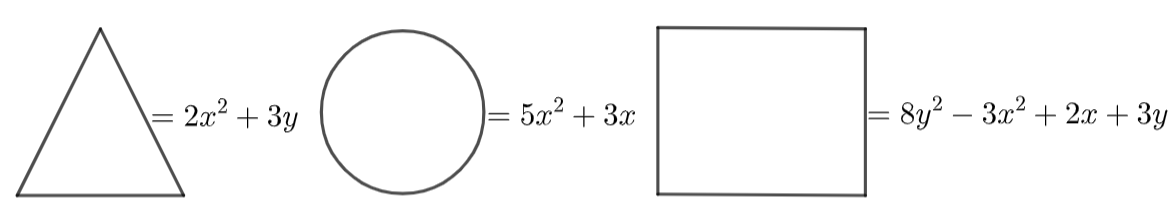The symbols are then represented in the expression:Ans: We need to substitute $2{x^2} + 3y$ in place of triangle figure, $5{x^2} + 3x$ in place of circle figure and $8{y^2} - 3{x^2} + 2x + 3y$ in place of square.

The symbols are then represented in the expression:

$\Rightarrow \left( {2{x^2} + 3y} \right) + \left( {5{x^2} + 3x} \right) - \left( {8{y^2} - 3{x^2} + 2x + 3y} \right)$

$\Rightarrow 2{x^2} + 3y + 5{x^2} + 3x - 8{y^2} + 3{x^2} - 2x - 3y$

$\Rightarrow 2{x^2} + 3{x^2} + 5{x^2} + 3y - 3y - 8{y^2} - 2x + 3x$

$\Rightarrow 10{x^2} - 8{y^2} + x$

73. Observe the following nutritional chart carefully:

 Food Item (Per Unit = 100g) Carbohydrates Rajma 60g Cabbage 5g Potato 22g Carrot 11g Tomato 4g Apples 14g

Write an algebraic expression for the amount of carbohydrates in ‘g’ for (a) y units of potatoes and 2 units of rajma

Ans: We can solve this using the unitary method.

1 unit of potato contains = 22 g carbohydrates.

Then y unit of potato contains = $22 \times y$ carbohydrates.

Similarly,

1 unit of rajma contains = 60 g carbohydrates

Then 2 units contain = $2 \times 60 = 120\,\,g$ carbohydrates.

Hence, the required expression is $22y + 120$.

(b) 2x units tomatoes and y units apples.

Ans: We can solve this using the unitary method.

2x unit of tomatoes contains = 4 g carbohydrates.

Then y unit of tomato potato contains = $2x \times 4$g carbohydrates.

Similarly,

1 unit of apple contains = 14 g carbohydrates

Then y unit contains = $14 \times y = 14y\,\,g$ carbohydrates.

Hence, the required expression is $8x + 14y$

74. Arjun bought a rectangular plot with length x and breadth y and then sold a triangular part of it whose base is y and height is z. Find the area of the remaining part of the plot.

Ans: Given,

Arjun bought a rectangular plot with length x and breadth y.

Then, Area of the rectangular plot =  length × breadth

$\Rightarrow x \times y = xy$

Now, given that triangular part of it whose base is y and height is z

Then the area of triangular part becomes

$\Rightarrow \dfrac{1}{2} \times y \times z$

$\Rightarrow \dfrac{1}{2}yz$

Therefore

Area of the remaining part of the plot = Area of the rectangular plot – Area of the triangular part.

$\Rightarrow$Remaining area $= xy - \dfrac{1}{2}yz$

$\Rightarrow$Remaining area $= y\left( {x - \dfrac{1}{2}z} \right)$

75. Amisha has a square plot of side m and another triangular plot with base and height each equal to m. What is the total area of both plots?

Ans: Given that the side length of the square plot is m.

Since it is a square all sides are equal.

Then the area of the square plot is = ${m^2}$

Height and base of the triangle plot is m then

The area of triangle plot $= \dfrac{1}{2}{m^2}$

Total area of the plot = area of the square plot + Area of the triangular plot.

$\Rightarrow$ Total area $= {m^2} + \dfrac{1}{2}{m^2}$

$\Rightarrow$ Total area $= \dfrac{3}{2}{m^2}$.

76. A taxi service charges Rupees 8km and levies a fixed charge of Rupees 50. Write an algebraic expression for the above situation, if the taxi is hired for x km.

Ans: Given, Taxi service charges Rs. 8 per km and fixed charge of Rs. 50.

If a taxi is hired for x km.

Then, algebraic expression for the situation

$\Rightarrow \left( {8 \times x} \right) + 50 = 8x + 50$

Hence, the required expression is $8x + 50$

77. Shiv works in a mall and gets paid Rs. 50 per hour. Last week he worked for 7 hours and this week he will work for x hours. Write an algebraic expression for the money paid to him for both the weeks.

Ans: Given that, Money paid to Shiv for some work is Rs. 50 per hour.

Money paid last week =Rs. 50 × 7 = Rs. 350

So, money paid this week = Rs. 50 × x = Rs. 50x

Total money paid to Shiv =Rs. (350 + 50x) =Rs. 50(x + 7)

78. Sonu and Raj have to collect different kinds of leaves for a science project. They go to a park where Sonu collects 12 leaves and Raj collects x leaves. After some time Sonu loses 3 leaves and Raj collects 2x leaves. Write an algebraic expression to find the total number of leaves collected by both of them.

Ans: According to the data,

Sonu collected leaves = 12 −3 = 9

Raj collected leaves = x + 2x = 3x

Total leaves collected = 9 + 3x

Hence, the required expression is 9+ 3x.

79. A school has a rectangular playground with length x and breadth y and a square lawn with side x as shown in the figure given below. What is the total perimeter of both of them combined together?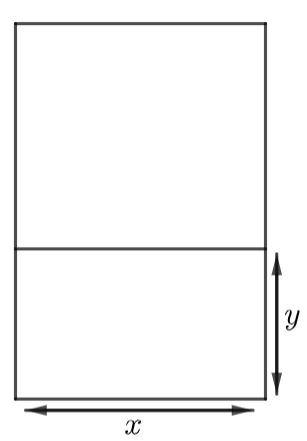Ans:

According to the given data,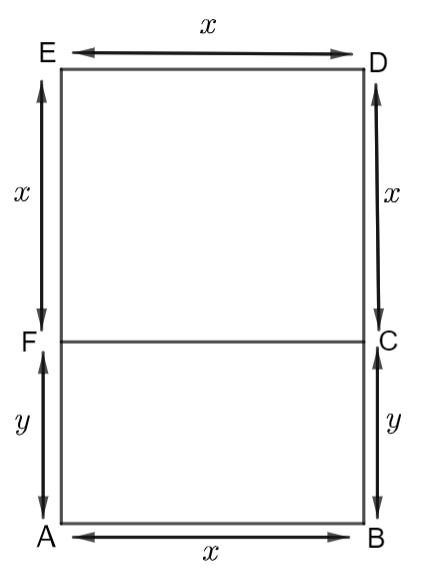Length of rectangular playground, AB = x

Breadth of rectangular playground, BC = y

Now, FCDE is a square, FC =CD =EF =DE = x

And ABCF is rectangle,

AB = FC = x BC = AF = y

Perimeter of any fig. is the sum of it’s all sides.

Perimeter of combine playground and lawn

= AB +BC +CD + DE + EF + FA

= x + y + x + x + x + y

= 4x + 2y

80. The rate of planting the grass is Rs. x square meter. Find the cost of planting the grass on a triangular lawn whose base is y meters and height is z meters.

Ans: We know that the area of the triangle is $\dfrac{1}{2} \times height \times base$.

Given that the base of the triangular lawn is y meters and the height of the triangular lawn is z meters.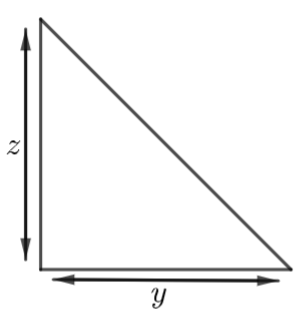Therefore, the area of triangular lawn

$\Rightarrow = \dfrac{1}{2} \times x \times z\,\,\,\,\,{m^2}$.

Now, the cost of planting the grass on lawn is

$\Rightarrow = \dfrac{1}{2}yz \times x$

$\Rightarrow Rs.\dfrac{1}{2}xyz$

81. Find the perimeter of the figure given below: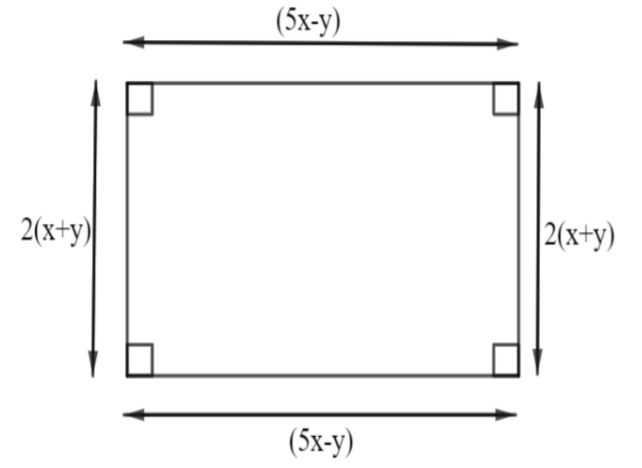Ans: We know that the perimeter of a rectangle is the sum of all 4 sides.

We know that perimeter = $2\left( {l + b} \right)$

Perimeter is given by

$\Rightarrow 2\left( {5x - y + 2\left( {x + y} \right)} \right)$

$\Rightarrow 2\left( {5x - y + 2x + 2y} \right)$

$\Rightarrow 2\left( {5x - y + 2x + 2y} \right)$

$\Rightarrow 2\left( {8x + y} \right)$

$\Rightarrow 14x + 2y$

82. In a rectangular plot, 5 square flower beds of side (x + 2) meters each have been laid (see figure given below). Find the total cost of fencing the flower beds at the Rs. 50 per cost of meters: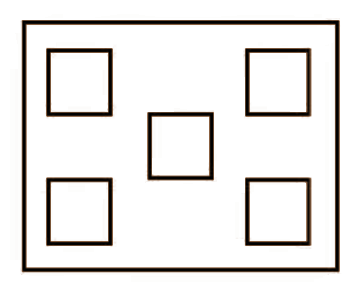Ans: Given,

The side of one square flower bed is (x + 2) meters.

Then we know that the perimeter of the square figure is four times its side.

That is perimeter of one square flower bed $= 4 \times side = 4\left( {x + 2} \right)\,$

Now there are 5 square flower beds.

Then the perimeter of these 5 square flower beds is given by

$= 5 \times 4\left( {x + 2} \right)\,$

$= 20\left( {x + 2} \right)\,\,meter$

Given that the cost of fencing of 100 m is Rs. 50

Then cost of 1 m is $= Rs.\dfrac{{50}}{{100}}$

Now the cost of $20\left( {x + 2} \right)\,\,meter$ is

$= 20\left( {x + 2} \right)\,\, \times Rs.\dfrac{{50}}{{100}}$

$= 20\left( {x + 2} \right)\,\, \times Rs.\dfrac{1}{2}$

$= Rs.10\left( {x + 2} \right)\,\,$

83. A wire is (7x – 3) meters long. A length of (3x – 4) meters is cut for use. Now, answer the following questions:

(a) How much wire is left?

Ans:

Given, length of wire = (7x – 3) meters long.

The wire cut for use has length = (3x – 4) m

Left wire = (7x – 3) – (3x – 4)

= 7x – 3 – 3x + 4

=7x – 3x – 3 + 4

= (4x + 1) m

(b) If this left out wire is used for making an equilateral triangle. What is the length of each side of the triangle so formed?

Ans:

Left wire = (4x +1) m

Perimeter of equilateral triangle = Length of wire left

$\Rightarrow 3 \times side = \left( {4x + 1} \right)$

$\Rightarrow side = \dfrac{{\left( {4x + 1} \right)}}{3}$

84. Rohan's mother gave him and his father gave him Out of the total money he spent on his birthday party. How much money is left with him?

Ans: Given that,

Amount given to Rohan by his mother = Rs.   $3{\kern 1pt} x{\kern 1pt} {y^2}$

And amount given to Rohan by his father = Rs.   $5(x{y^2} + 2)$

Total amount Rohan have =  $[(3x{y^2}) + (5x{y^2} + 10)]$

$= Rs.[3x{y^2} + 5x{y^2} + 10]$

$= Rs.(8x{y^2} + 10)$

Total amount spent by Rohan   $= Rs.(10 - 3x{y^2})$

After spending, Rohan have left money

$= Rs.(8x{y^2} + 10) - Rs.(10 - 3x{y^2})$

$= Rs.(8x{y^2} + 3x{y^2} + 10 - 10)$

$= Rs.10x{y^2}$

85. (i) A triangle is made up of 2 red sticks and 1 blue sticks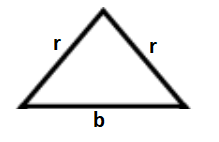. The length of a red stick is given by r and that of a blue stick is given by b. Using this information, write an expression for the total length of sticks in the pattern given below: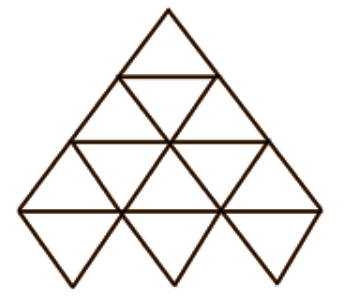Ans: Given, In a triangle,

Length of red stick = r

Length of blue stick = b

Now, calculate the number of red stick and blue stick in figure given in the question,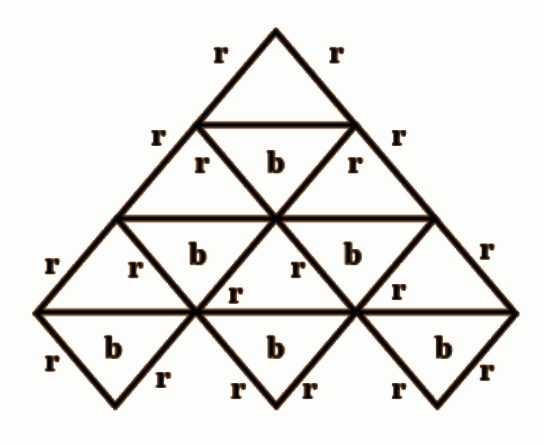The total number of red sticks = 18

The total number of blue sticks = 6

Then, total length of the sticks is   $18r + 6b$   or   $6\left( {3r + b} \right)$

(ii) In the given figure, the length of a green side is given by g and that of the red side is given by p.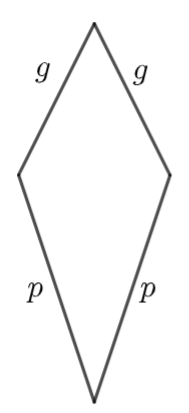Write an expression for the following pattern. Also write an expression if 100 such shapes are joined together.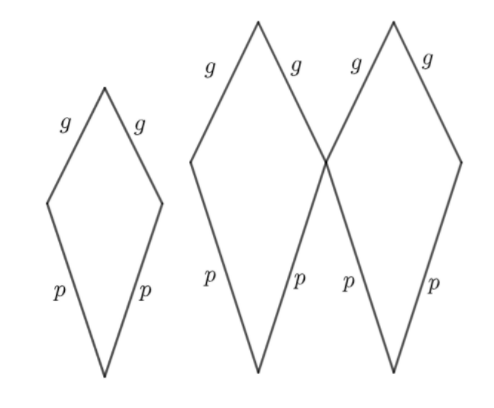Ans: Given,

Length of green side = g

Length of red side = p

From the figure given in the question,

The total length of one figure given in the question $\left( {2g + 2p} \right)$

According to the question, we have to write the expression for 100 shapes, then the required answer will be

$\Rightarrow 100\left( {2g + 2p} \right)$.

86. The sum of the first n natural numbers is given by $\dfrac{1}{2}{n^2} + \dfrac{1}{2}n$. Find
(i) The sum of the first 5 natural numbers.

Ans: To find the sum of first 5 natural number, put $n = 5$

$\Rightarrow \dfrac{1}{2}{\left( 5 \right)^2} + \dfrac{1}{2}\left( 5 \right)$

$\Rightarrow \dfrac{{25}}{2} + \dfrac{5}{2}$

$\Rightarrow \dfrac{{25 + 5}}{2}$

$\Rightarrow \dfrac{{30}}{2}$

$\Rightarrow 15$

(ii) The sum of the first 11 natural numbers.

Ans: To find the sum of first 5 natural number, put $n = 11$

$\Rightarrow \dfrac{1}{2}{\left( {11} \right)^2} + \dfrac{1}{2}\left( {11} \right)$

$\Rightarrow \dfrac{{121}}{2} + \dfrac{{11}}{2}$

$\Rightarrow \dfrac{{121 + 11}}{2}$

$\Rightarrow \dfrac{{132}}{2}$

$\Rightarrow 66$

(iii) The sum of natural numbers from 11 to 30

Ans: Sum of natural number from 11 to 30

= sum of first 30 natural numbers – sum of first 10 natural numbers.

$=\left[ \dfrac{1}{2}{{\left( 30 \right)}^{2}}+\dfrac{1}{2}\left( 30 \right) \right]-\left[ \dfrac{1}{2}{{\left( 10 \right)}^{2}}+\dfrac{1}{2}\left( 10 \right) \right]$

$=\left[ \dfrac{900}{2}+\dfrac{30}{2} \right]-\left[ \dfrac{100}{2}+\dfrac{10}{2} \right]$

$=\left[ 450+15 \right]-\left[ 50+5 \right]$

$=465-55$

$=410$

87. The sum of squares of first n natural numbers is given by $\dfrac{1}{6}n\left( {n + 1} \right)\left( {2n + 1} \right)$ or $\dfrac{1}{6}\left( {2{n^3} + 3{n^2} + n} \right)$. Find the sum of squares of the first 10 natural numbers.

Ans: Given the sum of squares of first n natural number is

$= \dfrac{1}{6}n\left( {n + 1} \right)\left( {2n + 1} \right)$

To find the sum of squares of first 10 natural number, put $n = 10$

$= \dfrac{1}{6}\left( {10} \right)\left( {10 + 1} \right)\left( {2\left( {10} \right) + 1} \right)$

$= \dfrac{1}{6}\left( {10} \right)\left( {11} \right)\left( {20 + 1} \right)$

$= \dfrac{1}{6} \times 10 \times 11 \times 21$

$= \dfrac{{2310}}{6}$

$= 385$

88. The sum of the multiplication table of natural numbers ‘n’ is given by 55 × n. Find the sum of

(a) Table of 7

Ans: Given that the sum of multiplication table of n natural number is $= 55 \times n$

Sum of table of 7 $= 55 \times 7 = 385$.

(b) Table of 10

Ans: Given that the sum of multiplication table of n natural number is $= 55 \times n$

Sum of table of 10 $= 55 \times 10 = 550$.

(c) Table of 19

Ans: Given that the sum of multiplication table of n natural number is $= 55 \times n$

Sum of table of 19 $= 55 \times 19 = 1045$.

89. If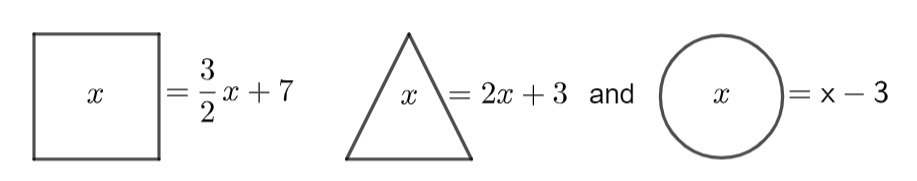Then find the value of:

(i)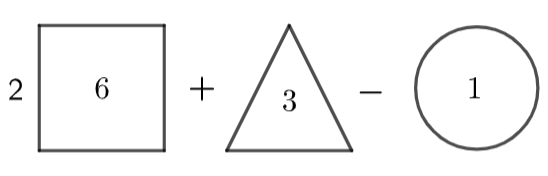Ans:

$= 2\left[ {\left( {2 \times 6} \right) + 3} \right] + \left[ {\left( {\dfrac{3}{2} \times 3} \right) + 7} \right] - \left[ {1 - 3} \right]$

$= 2\left[ {12 + 3} \right] + \left[ {\dfrac{9}{2} + 7} \right] - \left[ {1 - 3} \right]$

$= 2\left[ {15} \right] + \left[ {\dfrac{{9 + 14}}{2}} \right] - \left[ { - 2} \right]$

$= 30 + \dfrac{{23}}{2} + 2$

$= \dfrac{{60 + 23 + 4}}{2}$

$= \dfrac{{87}}{2}$

(ii)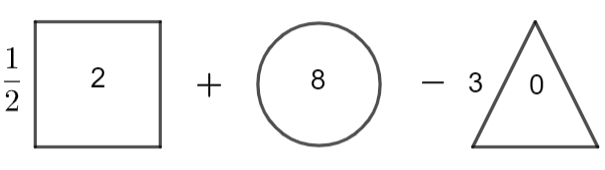Ans:

$= \dfrac{1}{2}\left[ {\left( {\dfrac{3}{2} \times 2} \right) + 7} \right] + \left[ {8 - 3} \right] - 3\left[ {\left( {2 \times 0} \right) + 3} \right]$

$= \dfrac{1}{2}\left[ {3 + 7} \right] + \left[ {8 - 3} \right] - 3\left[ {0 + 3} \right]$

$= \dfrac{1}{2}\left[ {10} \right] + \left[ 5 \right] - 3\left[ 3 \right]$

$= 5 + 5 - 9$

$= 1$

90. If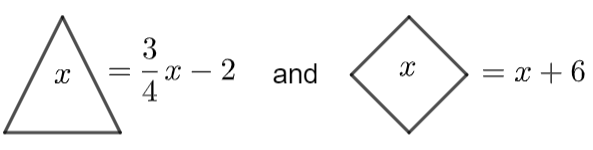, then find the value of

(i)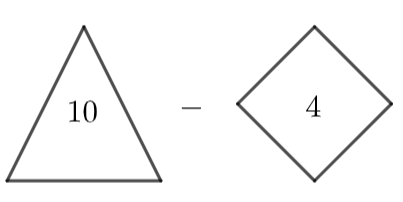Ans:

$= \left[ {\dfrac{3}{4} \times 10 - 2} \right] - \left[ {4 + 6} \right]$

$= \left[ {\dfrac{{30}}{4} - 2} \right] - \left[ {10} \right]$

$= \left[ {\dfrac{{30 - 8}}{4}} \right] - 10$

$= \left[ {\dfrac{{22}}{4}} \right] - 10$

$= \dfrac{{22 - 40}}{4}$

$= \dfrac{{ - 18}}{4}$

$= \dfrac{{ - 9}}{2}$

(ii)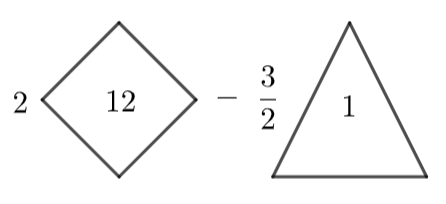Ans:

$= 2\left[ {12 + 6} \right] - \dfrac{3}{2}\left[ {\left( {\dfrac{3}{4} \times 1} \right) - 2} \right]$

$= 2\left[ {18} \right] - \dfrac{3}{2}\left[ {\dfrac{3}{4} - 2} \right]$

$= 36 - \dfrac{3}{2}\left[ {\dfrac{{3 - 8}}{4}} \right]$

$= 36 - \dfrac{3}{2}\left[ {\dfrac{{ - 5}}{4}} \right]$

$= 36 + \dfrac{{15}}{8}$

$= \dfrac{{288 + 15}}{8}$

$= \dfrac{{303}}{8}$

Translate each of the following algebraic expressions Question 91 to 94 into words.

91. $4b - 3$

Ans: Three subtracted from four times b.

92. $8\left( {m + 5} \right)$

Ans: Eight times the sum of m and 5.

93. $\dfrac{7}{{8 - x}}$

Ans: Quotient on dividing seven by the difference of eight and x.

94. $17\left( {\dfrac{{16}}{w}} \right)$

Ans: Seventeen times quotient of sixteen divided by w.

95. (i) Critical Thinking Write two different algebraic expressions for the word phrase “ $\left( {\dfrac{1}{4}} \right)$of the sum of x and 7.”

Ans: “ $\left( {\dfrac{1}{4}} \right)$of the sum of x and 7.”  Can be expressed as $\Rightarrow \dfrac{1}{4} \times \left( {x + 7} \right)$.

We know that addition is commutative.

So, we can express it as $\Rightarrow \dfrac{1}{4} \times \left( {7 + x} \right)$.

(ii) What’s the Error? A student wrote an algebraic expression for “5 less than a number n divided by 3” as $\dfrac{n}{3} - 5$. What error did the student make?

Ans: 5 less than a number n means  $\Rightarrow n - 5$

So, 5 less than a number n divided by 3 will be written $\Rightarrow \dfrac{{n - 5}}{3}$.

Students make an error of quotient.

(iii) Write About it Shashi used addition to solve a word problem about the weekly cost of commuting by toll tax for Rs. 15 each day. Ravi solved the same problem by multiplying. They both got the correct answer. How is this possible?

Total weekly cost is $\Rightarrow \left( {15 + 15 + 15 + 15 + 15 + 15 + 15} \right) = Rs.\,\,105$.

By multiplication method,

Total weekly cost = cost of one day $\times$ seven days

$= Rs.15 \times 7 = Rs.\,\,105$

96. Challenge Write an expression for the sum of 1 and twice a number n. If you let n be any odd number, will the result always be an odd number?

Ans: The sum of 1 and twice a number n can be written as

$\Rightarrow 1 + 2n$

If we put n= 3, which is a odd number

$\Rightarrow 1 + 2\left( 3 \right) = 1 + 6 = 7$

Yes, the result will always be an odd number.

97. Critical Thinking Will the value of 11x for x = –5 be greater than 11 or less than 11? Explain.

Ans: Given expression is 11x.

If we put x = -5, in the given expression.

$\Rightarrow 11\left( { - 5} \right) = - 55 < 11$

Hence, the value is less than 11.

98. Match Column I with Column II in the following:

 Column I Column II 1. The difference of 3 and a number squared a) $4 - 2x$ 2. 5 less than twice a number squared b) ${n^2} - 3$ 3. Five minus twice the square of a number c) $2{n^2} - 5$ 4. Four minus a number multiplied by 2 d) $5 - 2{n^2}$ 5. Seven times the sum of a number and 1 e) $3 - {n^2}$ 6. A number squared plus 6 f) $2\left( {n + 6} \right)$ 7. 2 times the sum of a number and 6 g) $7\left( {n + 1} \right)$ 8. Three less than the square of a number h) ${n^2} + 6$

Ans:

 Column I Column II 1. The difference of 3 and a number squared e) $3 - {n^2}$ 2. 5 less than twice a number squared c) $2{n^2} - 5$ 3. Five minus twice the square of a number d) $5 - 2{n^2}$ 4. Four minus a number multiplied by 2 a) $4 - 2x$ 5. Seven times the sum of a number and 1 g) $7\left( {n + 1} \right)$ 6. A number squared plus 6 h) ${n^2} + 6$ 7. 2 times the sum of a number and 6 f) $2\left( {n + 6} \right)$ 8. Three less than the square of a number b) ${n^2} - 3$

99. At the age of 2 years, a cat or a dog is considered 24 “human” years old. Each year, after age 2 is equivalent to 4 “human” years. Fill in the expression [24 ____ + (a – 2)] so that it represents the age of a cat or dog in human years. Also, you need to determine what ‘a’ stands for. Copy the chart and use your expression to complete it.

 Age [24 ____ + (a – 2)] Age (Human Years) 2 3 4 5 6

Ans:

According to the given questions, the expression is $24 + 4\left( {a - 2} \right)$ the age of cat or dog in human years, where a is present age of dog or cat.

 Age $24 + 4\left( {a - 2} \right)$ Age (Human Years) 2 $24 + 4\left( {2 - 2} \right)$ 24 3 $24 + 4\left( {3 - 2} \right)$ 28 4 $24 + 4\left( {4 - 2} \right)$ 32 5 $24 + 4\left( {5 - 2} \right)$ 36 6 $24 + 4\left( {6 - 2} \right)$ 40

100. Express the following properties with variables x, y and z.

Ans: Commutative property of addition is given by $\Rightarrow x + y = y + x$

(ii) Commutative property of multiplication

Ans: Commutative property of multiplication is given by $\Rightarrow x \times y = y \times x$.

Ans: Associative property of addition is given by $\Rightarrow x + \left( {y + z} \right) = \left( {x + y} \right) + z$

(iv) Associative property of multiplication

Ans: Associative property of multiplication is given by $\Rightarrow x \times \left( {y \times z} \right) = \left( {x \times y} \right) \times z$

(v) Distributive property of multiplication over addition

Ans: Distributive property of multiplication over addition is given by $\Rightarrow x \times \left( {y + z} \right) = \left( {x \times y} \right) + \left( {x \times z} \right)$

Vedantu offers the NCERT Exemplar for Class 7 Math Chapter - 10 Algebraic Expressions to the students which are completely free of cost. This NCERT Exemplar for Class 7 Math Chapter - 10 Algebraic Expressions is provided in PDF format, which can be downloaded by the students to study offline.

The subject matter experts at Vedantu had prepared these solutions: The NCERT Exemplar for Class 7 Math Chapter - 10 Algebraic Expressions appropriately. All these solutions are made concerning the latest NCERT syllabus and guidelines. These solutions are made to help and guide students and make them prepared for their examinations. These solutions assist students in their homework and assignments. Students can top the final exams and ace the subject by practicing these solutions.

## FAQs on NCERT Exemplar for Class 7 Maths Solutions Chapter 10 Algebraic Expressions

1: Are NCERT Exemplar for Class 7 Maths Chapter - 10 Algebraic Expressions

good to score better in Class 7 examinations?

Yes, NCERT Exemplar for Class 7 Maths Chapter - 10 Algebraic Expressions can help students to learn more and more of the subject and thus score better grades in the Class 7 examination. This is because, when students practice the solutions mentioned, they learn about all the concepts of the subject deeply. So they are well prepared for the examination. They don't need to be in chaos before the early days of the examination. Also, the learning power of the students is enhanced.

2: Where can I find useful study resources for NCERT Exemplar for Class 7 Maths Chapter - 10 Algebraic Expressions?

You can find NCERT Exemplar for Class 7 Maths Chapter - 10 Algebraic Expressions on the Vedantu app or website. You can find the NCERT Exemplar for Class 7 Maths Chapter - 10 Algebraic Expressions on the Vedantu site through the mentioned link

Vedantu offers these free resources which are created by the subject matter experts at Vedantu. They are masters in their respective fields and that's why the content is accurate and reliable. Students can find revision notes, important questions, question papers, etc on the site. These study materials are completely free and there is no cost involved. To access these useful study resources, all students have to sign in, and then they will be able to download what they want in pdf format online or offline.

3: Do you have to pay anything for studying NCERT Exemplar for Class 7 Maths Chapter - 10 Algebraic Expressions?

No, NCERT Exemplar for Class 7 Maths Chapter - 10 Algebraic Expressions is completely free of cost. Students can study it only by logging in to the Vedantu site which is absolutely free and just preparing for your examination. They don't need to pay anything to access this NCERT Exemplar for Class 7 Maths Chapter - 10 Algebraic Expressions. These are made for the convenience of the students. They are made not to loot students, but to make students prepare for the examination, to make them more adaptive, and make learning fun and enjoyable for them.

4: How much time does studying NCERT Exemplar for Class 7 Maths Chapter - 10 Algebraic Expressions involved?

Well it takes just some time to go through and understand these solutions. They are just made for the convenience of the students. It also depends upon students' memory power and intelligence. Some students can take less time to go through the revision notes while others can take more time also. It also depends upon the difficulty of the subjects and the chapter's concepts. Those with deeper concepts can be difficult to go through by the students while the normal easy-to-understand concepts and chapter revision notes can be easily gone through within a short time.

5: What is NCERT Exemplar?

NCERT Exemplars are practice books that include extra questions of a higher level. These NCERT Exemplars are made to help students in in-depth learning. The NCERT exemplar books contain conceptual sums, which cover CBSE board exams and competitive exams (JEE Main and JEE advanced). CBSE board exams sometimes contain in-depth sums.